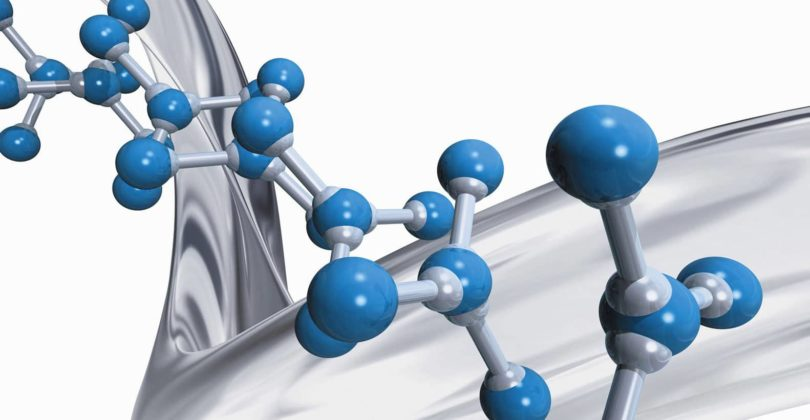# Chemical Bonding Questions Bank### Questions on Chemical Bonding for Practice:

Ques. The dipole moment is zero for the molecule
(a) Ammonia
(b) Boron trifluoride
(c) Sulphur dioxide
(d) water

Ans. (b)

Ques. Sodium chloride is an ionic compound whereas hydrogen chloride is a gas because
(a) Sodium is reactive
(b) Covalent bond is weaker than ionic bond
(c) Hydrogen chloride is a gas
(d) Covalent bond is stronger than ionic bond

Ans. (b)

Ques. According to Fajan’s rule, covalent bond is favoured by
(a) Large cation and small anion
(b) Large cation and large anion
(c) Small cation and large anion
(d) Small cation and small anion

Ans. (c)

Ques. The electronegativity of C, H, O, N and S are 2.5, 2.1, 3.5, 3.0 and 2.5 respectively. Which of the following bond is most polar
(a) O – H
(b) S – H
(c) N – H
(d) C – H

Ans. (a)

Ques. Number of sigma bonds in P4O10  is
(a) 6
(b) 7
(c) 17
(d) 16

Ans. (d)

Ques. A electrovalent compound is made up of
(a) Electrically charged molecules
(b) Neutral molecules
(c) Neutral atoms
(d) Electrically charged atoms or group of atoms

Ans. (d)

Ques. In the formation of NaCl from Na and Cl
(a) Sodium and chlorine both give electrons
(b) Sodium and chlorine both accept electrons
(c) Sodium loses electron and chlorine accepts electron
(d) Sodium accepts electron and chlorine loses electron

Ans. (c)

Ques. Percentage of s-character in sp3 hybrid orbital is
(a) 25
(b) 50
(c) 66
(d) 75

Ans. (a)

Ques. The main reaction during electrovalent bond formation is
(a) Redox reaction
(b) Substitution reaction
(d) Elimination reaction

Ans. (a)

Ques. Which one of the following statements is true for ammonium ion?
(a) All bonds are ionic
(b) All bonds are coordinate covalent
(c) H atoms are situated at the corners of a square
(d) H atoms are situated at the corners of a tetrahedron

Ans. (d)

Ques. Which among the following elements has the tendency to form covalent compounds?
(a) Ba
(b) Be
(c) Mg
(d) Ca

Ans. (b)

Related: Bones And Skeletal Tissues Quiz

Ques. The geometry of sulphur trioxide molecule is
(a) Tetrahedral
(b) Trigonal planar
(c) Pyramidal
(d) Square planar

Ans. (b)

Ques. The valency of phosphorus in H3PO4 is
(a) 2
(b) 5
(c) 4
(d) 1

Ans. (b)

Ques. As compared to pure atomic orbitals, hybrid orbitals have
(a) Low energy
(b) Same energy
(c) High energy
(d) None of these

Ans. (a)

Ques. The bond that exists between NH3 and BF3 is called
(a) Electrovalent
(b) Covalent
(c) Coordinate
(d) Hydrogen

Ans. (c)

Related: Boron family solved MCQ

Ques. The percentage s-character of the hybrid orbitals in methane, ethene and ethyne are respectively
(a) 25, 33, 50
(b) 25, 50, 75
(c) 50, 75, 100
(d) 10, 20, 40

Ans. (a)

Ques. Which of the following molecules will show dipole moment?
(a) Methane
(b) Carbon tetrachloride
(c) Chloroform
(d) Carbon dioxide

Ans. (c)

Ques. The solution of sugar in water contains
(a) Free atoms
(b) Free molecules
(c) Free ions
(d) Free atoms and free molecules

Ans. (b)

Ques. XeF2  molecule is
(a) Linear
(b) Triangular planar
(c) Pyramidal
(d) Square planar

Ans. (a)

Ques. Which one in the following contains ionic as well as covalent bond?
(a) CCl
(b) HCl
(c) KCN
(d) KCl

Ans. (c)